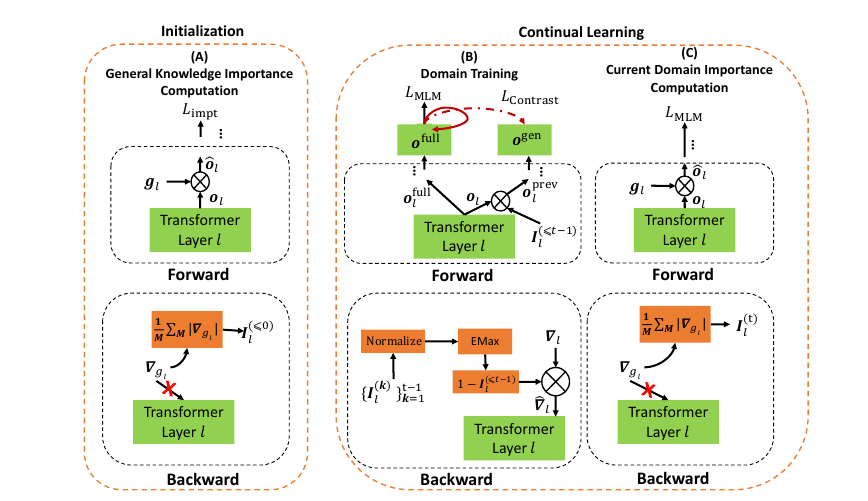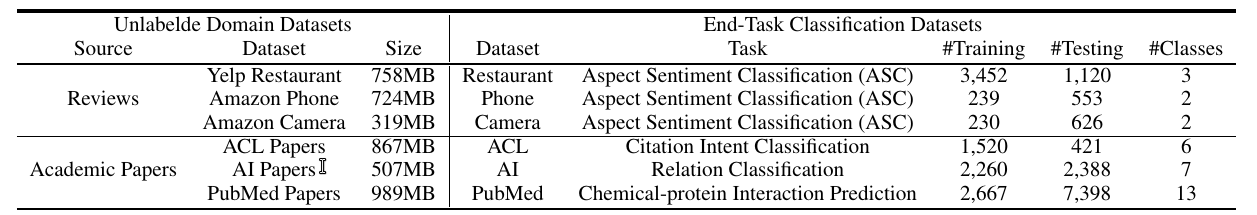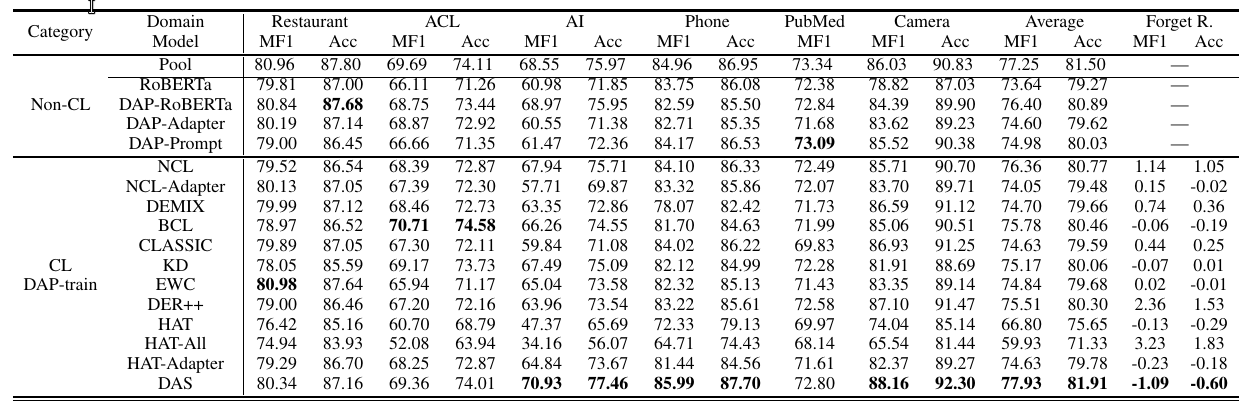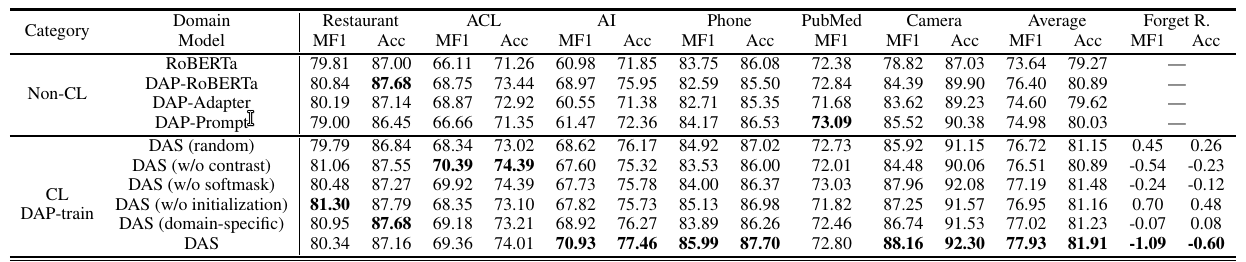# Continual Pre-training of Language Models论文解读

Posted by lili on November 13, 2023

## Introduction

### Contrastive Learning.

$L_{\text{contrast}} = - \frac{1}{N}\sum_{n=1}^N log \frac{e^{sim(q_n, q_n^+)/\tau}}{\sum_{j=1}^Ne^{sim(q_n, q_j^+)/\tau}}$

## DAS### 初始化

#### 一层中的重要单元

$\hat{o}_l = g_l \otimes o_l$

$I_l = \frac{1}{N} \sum_{n=1}^N | \frac{\partial \mathcal{L}_{\text{impt}}(x_n,y_n)}{\partial g_l} |$

#### 代理KL散度损失

$\mathcal{L}_{\text{impt}} = \text{KL}(f_{\text{LM}}^1(x_n^{\text{sub}},f_{\text{LM}}^2(x_n^{\text{sub}})$

#### 累计重要性

$I_l^{(\le t-1)} = \text{EMax}(I_l^{(t-1)}, I_l^{(\le t-2)})$

$\hat{\nabla_l} = (1-I_l^{(\le t-1)}) \otimes \nabla_l$

#### 对学到的知识和完整知识进行对比

$\mathcal{L}_{\text{contrast}} = - \frac{1}{N} \sum_{n=1}^N log \frac{e_n^{sim(o^{\text{full}}, o_n^{\text{full+}})/\tau}}{\sum_{j=1}^N(e^{sim(o_n^{\text{full}}, o_j^{\text{full+}})/\tau} + e^{sim(o_n^{\text{full}}, o_j^{\text{prev+}})/\tau})}$

【上式看起来有点复杂，其实思路很简单，就是让模型学习的方向是使得完整知识和之前知识差距尽量大，也就是要学新知识。】

#### 最终的损失函数

$\mathcal{L}_{\text{DAP-train}} = \mathcal{L}_{\text{MLM}} + \lambda \mathcal{L}_{\text{contrast}}$

## 实验

### 数据集和baseline

#### 数据集#### baseline

##### 持续学习

ELLE未包含在内，因为我们通过从RoBERTa学习来适应它，但它未能收敛。

### 结果分析和消融实验

#### 终端任务结果(2) DAS既实现了防止遗忘，又实现了知识迁移。那些只关注遗忘防止的基线（KD、EWC、DER++）在性能上较差，因为它们为了避免对抗性遗忘而牺牲了准确性。那些关注知识迁移的基线（BCL、CLASSIC和DEMIX）取得了更好的结果，但仍然比DAS差。DEMIX的知识迁移效果很弱。BCL在避免对抗性遗忘的同时实现了一些知识迁移，但比NCL更弱。总体而言，CL基线的性能都较差，因为它们缺乏鼓励知识迁移的方法，或者必须依赖于适配器。

(4）使用完整的语言模型学习所有任务，而不是使用子网络（基于HAT方法）使DAS更为有效。HAT的表现较差，表明它不适用于DAP训练，正如在第1节中讨论的那样。即使我们使用所有特征（不仅仅是相应子网络中的特征），我们仍然得到了差的结果（HAT-All），因为在DAP训练中使用的特征（在语言模型子网络中）与在终端任务微调中使用的特征（来自整个语言模型的特征）不同。

#### KL散度代理损失的有效性

##### 和$D_0$的采样子集对比#### 消融实验